Courses

# Test: Syntax Directed Translation- 2

## 9 Questions MCQ Test Question Bank for GATE Computer Science Engineering | Test: Syntax Directed Translation- 2

Description
This mock test of Test: Syntax Directed Translation- 2 for Computer Science Engineering (CSE) helps you for every Computer Science Engineering (CSE) entrance exam. This contains 9 Multiple Choice Questions for Computer Science Engineering (CSE) Test: Syntax Directed Translation- 2 (mcq) to study with solutions a complete question bank. The solved questions answers in this Test: Syntax Directed Translation- 2 quiz give you a good mix of easy questions and tough questions. Computer Science Engineering (CSE) students definitely take this Test: Syntax Directed Translation- 2 exercise for a better result in the exam. You can find other Test: Syntax Directed Translation- 2 extra questions, long questions & short questions for Computer Science Engineering (CSE) on EduRev as well by searching above.
QUESTION: 1

Solution:
QUESTION: 2

### Consider the following procedure declaration: procedure p ; x : integer; procedure q; begin x = x + 1 end; procedure r; x : integer; begin x := 1; q ; write(x) end; begin x = 2; r; end; The output produced by calling P in a language with static scope will be

Solution:

Since value output by program is the value of x which is declared in side DC function, so, output is 1 using static scoping.

QUESTION: 3

### Consider the following procedure declaration: procedure p ; x : integer; procedure q; begin x = x + 1 end; procedure r; x : integer; begin x := 1; q ; write(x) end; begin x = 2; r; end; The output produced by calling Pin a language with dynamic scope will be

Solution:

Since value output by program is the value of x which is declared in side DC function, so, output is 1 using dynamic scoping.

QUESTION: 4

Choose the false statements:
1. Control stack keeps track of live procedure activations.
2. Activation records can be managed with the help of stack.
3. Dangling reference is a reference to a storage that has been deallocated

Solution:
• Control stack keeps tracks of live procedure.
• Activation records can be managed with.
• Dangling reference is a reference to a storage that has been de-allocated.

All statement are true.

QUESTION: 5

'Divide by Zero ’ is a

Solution:

It is a semantic error (run-time error).

QUESTION: 6

Consider the following translation scheme: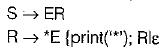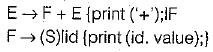Here id is a token that represents an integer and id.value represents the corresponding integer value. For an input ‘2 * 3 + 4 ’ , this translation scheme prints

Solution: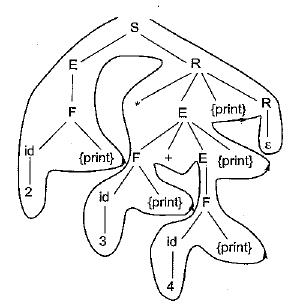So an input 2 * 3 + 4, it prints from the above parse tree as 234 + *.

QUESTION: 7

Consider the following Syntax Directed Translation Scheme (SDTS), with non-terminals {S, A} and terminals {a, b }.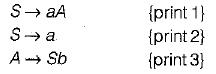Using the above SDTS, the output printed by a bottom-up parser, for the input aab is:

Solution:

Input is ‘aab’
So tree for given input.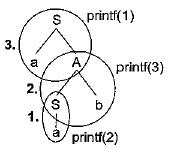So output will be 2, 3 and 1 because printed order will be 1, 2, 3.
So output: 2 3 1

QUESTION: 8

What is printed by the print statements in the program P1 assuming call by reference parameter passing?
Program P1 ()
{i
x = 10;
y= 3;
func (y, x , x);
print x;
print y;
}
func1 (x, y, z)
y = y + 4;
z = x + y + z;

Solution:

Actual output will be x = 31 and y = 3.

QUESTION: 9

Consider the following statements:

S1 Static allocation bindings do not change at runtime.
S2: Heap allocation allocates and de-allocates storage at run time.
Which of the above statements is/are true

Solution:
• Static allocation binding do not change at runtime.
• Heap allocation allocated and deallocate storage at run time